# Oscillatory MHD Mass Transfer Channel Flow in a Rotating System with Hall Current

Oscillatory MHD Mass Transfer Channel Flow in a Rotating System with Hall Current

N. Ahmed S. M. Das*

Department of Mathematics, Gauhati University, Guwahati 781014, Assam, India

Corresponding Author Email:
suwagmoni1.618@gmail.com
Page:
115-123
|
DOI:
https://doi.org/10.18280/ijht.340117
|
Accepted:
|
Published:
31 March 2016
| Citation

OPEN ACCESS

Abstract:

The effects of heat and mass transfer on the flow of an oscillatory convective MHD channelflow of an electrically conducting viscous incompressible fluid in a slip flow regime in the presence of Hall current and thermal radiation are studied. A magnetic field of uniform strength is applied normal to the plates of channel. The two vertical porous plates of the channel are subjected to a constant injection/suction. The entire system rotates about an axis normal to the plates with a uniform angular velocity $\vec{\Omega}$. A closed form analytical solutions to the equations governing the fluid motion are obtained for the velocity, temperature and concentration fields. The numerical calculations for the velocity, temperature and concentration fields, and the coefficient of skin friction, rate of heat and mass transfer at the plates are performed. The results are presented graphically for different values of the physical parameters involved. It is seen that primary fluid flow is retarded due to angular velocity of the system.

Keywords:

Convective flow, Hall current, Rotating channel, Slip conditions, Thermal radiation.

1. Introduction

The phenomenon of heat and mass transfer has been the object of extensive research due to its application in science and technology. Such phenomenon is observed in buoyancy induced motions in the atmosphere, in the bodies of water, quasi-solid bodies such as earth and so on. Oscillatory flows are associated with higher rates of heat and mass transfer. Many studies have been done to understand its characteristics in different systems such as reciprocating engines, pulse combustors and chemical reactors etc. Unsteady oscillatory free convective flows play an importance in aerospace technology, turbo machinery and chemical engineering. Such flows arise due to either unsteady motion of a boundary or boundary temperature. In nature and industrial applications many transport processes exist where the transfer of heat and mass takes place simultaneously as a result of combined buoyancy effects of thermal diffusion and diffusion of chemical species. Cooper et al.  has made a detailed study on fluid mechanics of oscillatory and modulated flows and associated applications in heat and mass transfer. Muthucumaraswamy  has studied the effect of heat and mass transfer on flow past an oscillatory vertical plate with variable temperature. Ahmed & Kalita  discussed the combined heat mass transfer effects on Oscillatory MHD free and forced convection flow through a porous medium in presence of a heat source with variable suction. The hydrodynamic rotating flow of an electrically conducting viscous incompressible fluid has gained considerable attention because of its numerous applications in physics and engineering. In geophysics, it is applied to measure and study the positions and velocities with respect to a fixed frame of reference on the earth, which rotates with respect to an inertial frame in the presence of its magnetic field. Boundary layer flows in a rotating fluid system are important due to various applications in science and technology. Debnath  presented exact solutions of the hydrodynamic and hydro magnetic boundary layer equations in such systems. Oscillatory hydro magnetic Couette flow in a rotating fluid system is investigated by Guria et al. 

When the density of an electrically conducting fluid is low and/or applied magnetic field is strong, a current is induced in a direction which is normal to both the electric and magnetic fields. Thus if an electric field is applied at right angle to the magnetic field, the total current will not flow along the electric field. This tendency of the electric current to flow across an electric field in the presence of a magnetic field is called Hall effects and the resulting current is known as Hall current. Hall effects are important when the Hall parameter, which is the ratio between the electron-cyclotron frequency and the electron-atom-collision frequency, is high. This happens when the magnetic field is strong or when the collision frequency is low. Hall currents are of great importance in many astrophysical problems, Hall accelerator and flight MHD as well as flows of plasma in a MHD power generator. Aboeldahab and Elbarbary  considered effects of Hall current on MHD free convection flow with heat and mass transfer over a vertical in the presence of a strong external magnetic field. Sarkar et al  investigated effects of Hall currents and radiation on MHD free convection flow past an oscillating vertical plate with oscillatory plate temperature in a porous medium.

It is assumed that the boundary condition for a viscous fluid at a solid surface is one of “no - slip” at the macroscopic level. While no – slip boundary condition has been proven experimentally to be accurate for a number of macroscopic flows, it remains an assumption that is based on physical principles. In fact nearly two hundred years ago, Navier’s  proposes a general a boundary condition that incorporates the possibility of the fluid slip at a solid boundary. Navier’s proposed condition assumes that the fluid slip velocity at a solid surface is proportional to the shear stress at the surface. The mathematical form of the Navier’s proposed condition on the slip velocity as emphasized by Goldstein  is $u=\gamma\left(\frac{\partial u}{\partial \eta}\right)$; where $\gamma$ is the slip strength or slip coefficient, u is the slip velocity and $\eta$ is the normal coordinate. If $\gamma=0$ then no-slip boundary condition is obtained. If $\gamma$ is finite, fluid slip occurs at the wall but its effect depends upon the length scale of the flow. The effect of slip condition on MHD steady flow in a channel with permeable boundaries has been discussed by Makinde and Osalusi . Marques et al.  has considered the effect of fluid slippage at the plate for Couette flow. Ahmed and Kalita  have studied MHD oscillatory free convective flow past a vertical plate in slip-flow regime with variable suction and periodic plate temperature

Recently Singh and Pathak  have studied the effects of Hall current and thermal radiation on MHD convective flow in a vertical rotating porous channel in slip flow regime in presence of uniform magnetic field where the entire system rotates about the axis perpendicular to the planes of the plates of the channel.

The method of the present work is similar to the work of Singh and Pathak  although the flow geometries are not identical. Further in the present work the effect of mass transfer is taken into account which was not considered in the work of Singh and Pathak .

The objective of the present work is to investigate the effects of Hall current and thermal radiation on MHD oscillatory convective mass transfer flow through a rectangular channel bounded by two vertical porous plates in a rotating system with slip flow regime.

2. Mathematical Modelof The Problem

The equations governing the unsteady motion of an incompressible, viscous, electrically conducting fluid with a vertical channel system in presence of magnetic field areas follows:

Continuity equation:

$\vec{\nabla} \cdot \overrightarrow{\mathrm{V}}=0$(1)

Gauss’s law of magnetism:

$\vec{\nabla} \cdot \overrightarrow{\mathrm{B}}=0$(2)

Kirchhoff’s first law:

$\vec{\nabla} \cdot \overrightarrow{\mathrm{J}}=0$(3)

General Ohm’s law:

$\overrightarrow{\mathrm{J}}+\frac{\omega_{\mathrm{e}} \tau_{\mathrm{e}}}{\mathrm{B}}(\overrightarrow{\mathrm{J}} \times \overrightarrow{\mathrm{B}})=\sigma\left[\overrightarrow{\mathrm{E}}+\overrightarrow{\mathrm{V}} \times \overrightarrow{\mathrm{B}}+\frac{1}{\mathrm{e} \eta_{\mathrm{e}}} \nabla \mathrm{p}_{\mathrm{e}}\right]$(4)

Momentum equation:

\begin{aligned} \rho\left[\frac{\partial \overrightarrow{\mathrm{V}}}{\partial t^{\prime}}+\vec{\Omega} \times \overrightarrow{\mathrm{V}}+(\overrightarrow{\mathrm{V}} \overrightarrow{\mathrm{P}}) \overrightarrow{\mathrm{V}}\right] &=-\vec{\nabla} \mathrm{p}+\overrightarrow{\mathrm{J}} \times \overrightarrow{\mathrm{B}}+\mu \nabla^{2} \overrightarrow{\mathrm{V}} \\ &+\mathrm{g} \beta \mathrm{T}^{\prime}+\mathrm{g} \overline{\beta} \mathrm{C}^{\prime} \end{aligned}(5)

Energy equation:

$\rho \mathrm{C}_{\mathrm{p}}\left[\frac{\partial \mathrm{T}^{\prime}}{\partial \mathrm{t}^{\prime}}+(\overrightarrow{\mathrm{V}} \cdot \vec{\nabla}) \mathrm{T}^{\prime}\right]=\mathrm{k} \nabla^{2} \mathrm{T}^{\prime}-\vec{\nabla} \cdot \mathrm{q}$(6)

Species continuity equation:

$\frac{\partial C^{\prime}}{\partial t^{\prime}}+(\vec{V} \cdot \vec{\nabla}) C^{\prime}=D \nabla^{2} C^{\prime}$(7)

We now consider an oscillatory MHD convective flow of an electrically conducting viscous incompressible fluid through a channel bounded by two infinite vertical porous plate separated by distance‘d’, taking into account the Hall current. One of the plates of the channel is subjected to slip velocity. The $X^{\prime}$ axis is taken oriented vertically upward along the centreline of the channel, the $\mathrm{Z}^{\prime}$ axis is taken normal to the planes to the plates which is the axis of rotation and $\mathrm{Y}^{\prime}$ is taken along the width of the either plate.

## figure_1.png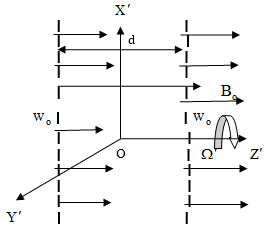Figure 1. Physical model of the problem

In order to make the mathematical model of the present work idealized, the present investigation is restricted the following assumptions:

1. All the fluid properties are considered constant except the influence of the density with temperature and concentration in the buoyancy force.
2. The viscous and Ohmic dissipations of energy are negligible.
3. The magnetic Reynolds number is small that the induced magnetic field can be neglected.
4. The plate is electrically insulator and the plate temperature as well as the plate concentration varies linearly as the distance along the plate.
5. No external electric field is applied for which the polarization voltage is negligible leading to $\overrightarrow{\mathrm{E}}=\overrightarrow{0}$

Let $\overrightarrow{\mathrm{V}}=\left(\mathrm{u}^{\prime}, \mathrm{V}^{\prime}, \mathrm{W}^{\prime}\right)$ denote the fluid velocity,$\vec{\Omega}=\left(0,0, \Omega^{\prime}\right)$ be the angular velocity,$\vec{B}=\left(0,0, B_{o}\right)$ be the magnetic flux density and $\overrightarrow{\mathrm{J}}=\left(\dot{\mathrm{j}}_{\mathrm{x}^{\prime}}, \dot{\mathrm{j}}_{\mathrm{y}^{\prime}}, \dot{\mathrm{J}}_{\mathrm{z}^{\prime}}\right)$ be the current density at the point $P\left(x^{\prime}, y^{\prime}, z^{\prime}, t^{\prime}\right)$ in the fluid. As the plates of the channel are infinite in $X^{\prime}$ and $Y^{\prime}$ directions, therefore all the physical quantities are independent of $x^{\prime}$ and $y^{\prime}$ .

Equation (1) yields

\begin{aligned} \frac{\partial \mathrm{u}^{\prime}}{\partial \mathrm{x}^{\prime}}+\frac{\partial \mathrm{v}^{\prime}}{\partial \mathrm{y}^{\prime}} &+\frac{\partial \mathrm{w}^{\prime}}{\partial \mathrm{z}^{\prime}}=0 \\ \Rightarrow \frac{\partial \mathrm{w}^{\prime}}{\partial \mathrm{z}^{\prime}} &=0 \end{aligned}

which holds for $\mathrm{w}^{\prime}=\mathrm{w}_{\mathrm{o}}$ (constant)

The equation (2) is trivially satisfied by the magnetic flux density distribution $\overrightarrow{\mathrm{B}}=\left(0,0, \mathrm{B}_{\mathrm{o}}\right)$

The equation (3) gives, $\frac{\partial \mathrm{j}_{z^{\prime}}}{\partial \mathrm{z}^{\prime}}=0$ , which is satisfied with $j_{z^{\prime}}$ is constant.

Further, as the plates are electrically insulators, so $j_{z^{\prime}}=0$  on the walls and, hence $j_{z^{\prime}}=0$  for the entire fields.

Again on the basis of the above assumptions, equation (4) takes the form

$\vec{J}+\frac{\omega_{e} \tau_{e}}{B}(\vec{J} \times \vec{B})=\sigma(\vec{V} \times \vec{B})$(8)

Splitting the equation (8), we derive the following equations

$j_{x^{\prime}}+m j_{y^{\prime}}-\sigma B_{o} v^{\prime}=0$(i)

$-\mathrm{m} \mathrm{j}_{\mathrm{x}^{\prime}}+\mathrm{j}_{\mathrm{y}^{\prime}}+\sigma \mathrm{B}_{\mathrm{o}} \mathrm{u}^{\prime}=0$(ii)

Solving (i) and (ii), we obtain

$\mathrm{j}_{\mathrm{x}^{\prime}}=\frac{\sigma \mathrm{B}_{\mathrm{o}}\left(\mathrm{mu}^{\prime}+\mathrm{v}^{\prime}\right)}{1+\mathrm{m}^{2}}$  and $\mathrm{j}_{\mathrm{y}^{\prime}}=\frac{\sigma \mathrm{B}_{\mathrm{o}}\left(\mathrm{mv}^{\prime}-\mathrm{u}^{\prime}\right)}{1+\mathrm{m}^{2}}$

where $\mathrm{m}=\omega_{\mathrm{e}} \tau_{\mathrm{e}}$ is the Hall parameter.

Under the above assumptions, the governing equations (5), (6) and (7) reduces to

\begin{aligned} \frac{\partial u^{\prime}}{\partial t^{\prime}}+w_{o} \frac{\partial u^{\prime}}{\partial z^{\prime}}=&-\frac{1}{\rho} \frac{\partial p^{\prime}}{\partial x^{\prime}}+v \frac{\partial^{2} u^{\prime}}{\partial z^{\prime 2}}+2 \Omega^{\prime} v^{\prime}+\frac{\sigma B_{o}^{2}\left(m v^{\prime}-u^{\prime}\right)}{\rho\left(1+m^{2}\right)} \\ &+g \beta\left(T^{\prime}-T_{o}\right)+g \overline{\beta}\left(C^{\prime}-C_{o}\right) \end{aligned}(9)

$\frac{\partial v^{\prime}}{\partial t^{\prime}}+w_{o} \frac{\partial v^{\prime}}{\partial z^{\prime}}=-\frac{1}{\rho} \frac{\partial p^{\prime}}{\partial y^{\prime}}+v \frac{\partial^{2} v^{\prime}}{\partial z^{\prime 2}}-2 \Omega^{\prime} u^{\prime}-\frac{\sigma B_{o}^{2}\left(m u^{\prime}+v^{\prime}\right)}{\rho\left(1+m^{2}\right)}$(10)

$\rho \mathrm{C}_{\mathrm{p}}\left(\frac{\partial \mathrm{T}^{\prime}}{\partial \mathrm{t}^{\prime}}+\mathrm{w}_{\mathrm{o}} \frac{\partial \mathrm{T}^{\prime}}{\partial \mathrm{z}^{\prime}}\right)=\mathrm{k} \frac{\partial^{2} \mathrm{T}^{\prime}}{\partial\mathrm{z}^{\prime 2}}-\frac{\partial \mathrm{q}}{\partial \mathrm{z}^{\prime}}$(11)

$\frac{\partial C^{\prime}}{\partial t^{\prime}}+w_{\circ} \frac{\partial C^{\prime}}{\partial z^{\prime}}=D \frac{\partial^{2} C^{\prime}}{\partial z^{\prime 2}}$(12)

The last term in the energy equation (11) stands for radiative heat flux is given by

$\frac{\partial \mathrm{q}}{\partial \mathrm{z}^{\prime}}=4 \alpha^{2}\left(\mathrm{T}^{\prime}-\mathrm{T}_{\mathrm{o}}\right)$(13)

where $\alpha$  is the mean radiation absorption coefficient

The appropriate boundary and slip flow conditions for the present problem are

$\mathrm{u}^{\prime}=\mathrm{L} \frac{\partial \mathrm{u}^{\prime}}{\partial \mathrm{z}^{\prime}}, \quad \mathrm{v}^{\prime}=\mathrm{L} \frac{\partial \mathrm{v}^{\prime}}{\partial \mathrm{z}^{\prime}}, \quad \mathrm{T}^{\prime}=\mathrm{T}_{\mathrm{o}}, \quad \mathrm{C}^{\prime}=\mathrm{C}_{\mathrm{o}} \quad$ at $\quad \mathrm{z}^{\prime}=\frac{-\mathrm{d}}{2}$

$u^{\prime}=v^{\prime}=0$ , $\mathrm{T}^{\prime}=\mathrm{T}_{\mathrm{o}}\left(1+\cos \omega^{\prime} \mathrm{t}^{\prime}\right)$ , $C^{\prime}=C_{o}\left(1+\cos \omega^{\prime} t^{\prime}\right)$  at

$z^{\prime}=\frac{d}{2}$(14)

where L is the mean free path which is constant for an incompressible fluid.

The non dimensional quantities are

\eta=\frac{z^{\prime}}{d}, \mathrm{x}=\frac{\mathrm{x}^{\prime}}{\mathrm{d}}, \quad \mathrm{y}=\frac{\mathrm{y}^{\prime}}{\mathrm{d}}, \quad \mathrm{u}=\frac{\mathrm{u}^{\prime}}{\mathrm{U}}, \quad v=\frac{v^{\prime}}{d}, \quad \mathrm{T}=\frac{\mathrm{T}^{\prime}-\mathrm{T}_{0}}{\mathrm{T}_{0}}C=\frac{C^{\prime}-C_{o}}{C_{o}}, t=\frac{t^{\prime} U}{d}, \omega=\frac{\omega^{\prime} \mathrm{d}}{\mathrm{U}}, \mathrm{p}=\frac{\mathrm{p}^{\prime}}{\rho \mathrm{U}^{2}} (15) With the help of non dimensional quantities (15), equations (9) to (12) reduces to \begin{aligned} \frac{\partial \mathrm{u}}{\partial \mathrm{t}}+\frac{\lambda}{\mathrm{R}_{\mathrm{e}}} \frac{\partial \mathrm{u}}{\partial \eta}=&-\frac{\partial \mathrm{p}}{\partial \mathrm{x}}+\frac{1}{\mathrm{R}_{\mathrm{e}}} \frac{\partial^{2} \mathrm{u}}{\partial \eta^{2}}+\frac{2 \Omega}{\mathrm{R}_{\mathrm{e}}} \mathrm{v}+\frac{\mathrm{M}^{2}(\mathrm{mv}-\mathrm{u})}{\mathrm{R}_{\mathrm{e}}\left(1+\mathrm{m}^{2}\right)} \\ &+\frac{\mathrm{G}_{\mathrm{r}}}{\mathrm{R}_{\mathrm{e}}}\mathrm{T}+\frac{\mathrm{G}_{\mathrm{m}}}{\mathrm{R}_{\mathrm{e}}} \mathrm{C} \end{aligned}(16) \frac{\partial \mathrm{v}}{\partial \mathrm{t}}+\frac{\lambda}{\mathrm{R}_{\mathrm{e}}} \frac{\partial \mathrm{v}}{\partial \eta}=-\frac{\partial \mathrm{p}}{\partial \mathrm{y}}+\frac{1}{\mathrm{R}_{\mathrm{e}}} \frac{\partial^{2} \mathrm{v}}{\partial \eta^{2}}-\frac{2 \Omega}{\mathrm{R}_{\mathrm{e}}} \mathrm{u}-\frac{\mathrm{M}^{2}(\mathrm{mu}+\mathrm{v})}{\mathrm{R}_{\mathrm{e}}\left(1+\mathrm{m}^{2}\right)}(17) \frac{\partial \mathrm{T}}{\partial \mathrm{t}}+\frac{\lambda}{\mathrm{R}_{\mathrm{e}}} \frac{\partial \mathrm{T}}{\partial \mathrm{n}}=\frac{1}{\mathrm{P}_{\mathrm{r}} \mathrm{R}_{\mathrm{e}}} \frac{\partial^{2} \mathrm{T}}{\partial \eta^{2}}-\frac{\mathrm{N}^{2}}{\mathrm{P}_{\mathrm{r}} \mathrm{R}_{\mathrm{e}}} \mathrm{T}(18) \frac{\partial \mathrm{C}}{\partial \mathrm{t}}+\frac{\lambda}{\mathrm{R}_{\mathrm{e}}} \frac{\partial \mathrm{C}}{\partial \eta}=\frac{1}{\mathrm{S}_{\mathrm{c}} \mathrm{R}_{\mathrm{e}}} \frac{\partial^{2} \mathrm{C}}{\partial \eta^{2}}(19) where, \mathrm{R}_{\mathrm{e}}=\frac{\mathrm{Ud}}{\mathrm{v}}, \Omega=\frac{\Omega^{\prime} d^{2}}{v}, \lambda=\frac{w_{o} d}{v}, G_{r}=\frac{g \beta d^{2} T_{o}}{v U} \mathrm{G}_{\mathrm{m}}=\frac{\mathrm{g} \overline{\beta} \mathrm{d}^{2} \mathrm{C}_{\mathrm{o}}}{\mathrm{v} \mathrm{U}}, \mathrm{P}_{\mathrm{r}}=\frac{\mu \mathrm{C}_{\mathrm{p}}}{\mathrm{k}}, \mathrm{S}_{\mathrm{c}}=\frac{\mathrm{v}}{\mathrm{D}} N=\frac{2 \alpha d}{\sqrt{k}}, M=B_{o} d \sqrt{\frac{\sigma}{\rho v}} All the physical quantities are defined in nomenclature. The boundary conditions in the non-dimensional form becomes \begin{array}{ll}{\eta=-\frac{1}{2} :} & {\mathrm{u}=\mathrm{h} \frac{\partial \mathrm{u}}{\partial \eta}, \mathrm{v}=\mathrm{h} \frac{\partial \mathrm{v}}{\partial \eta}, \mathrm{T}=0, \quad \mathrm{C}=0} \\ {\eta=\frac{1}{2} :} & {\mathrm{u}=\mathrm{v}=0, \quad \mathrm{T}=\cos \omega \mathrm{t}, \mathrm{C}=\cos \omega \mathrm{t}}\end{array}(20) where \mathrm{h}=\frac{\mathrm{L}}{\mathrm{d}} is the slip flow parameter. We assume that the fluid flow under the influence of a non-dimensional oscillatory pressure gradient varying periodically with the time in the direction of x-axis of the following form -\frac{\partial p}{\partial x}=P \cos \omega t 3. Solution of The Problem We introduce a complex velocity function of the form F(\eta, t)=u(\eta, t)+i v(\eta, t)(21) Equations (16) and (17) can be combined to give \begin{aligned} \frac{\partial \mathrm{F}}{\partial \mathrm{t}}+\frac{\lambda}{\mathrm{R}_{\mathrm{e}}} \frac{\partial \mathrm{F}}{\partial \eta}=& \mathrm{P} \cos \omega \mathrm{t}+\frac{1}{\mathrm{R}_{\mathrm{e}}} \frac{\partial^{2} \mathrm{F}}{\partial \eta^{2}}-\frac{2 \mathrm{i} \Omega}{\mathrm{R}_{\mathrm{e}}} \mathrm{F}-\frac{\mathrm{M}^{2}(1+\mathrm{im})}{\mathrm{R}_{\mathrm{e}}\left(1+\mathrm{m}^{2}\right)} \mathrm{F} \\ &+\frac{\mathrm{G}_{\mathrm{r}}}{\mathrm{R}_{\mathrm{e}}}\mathrm{T}+\frac{\mathrm{G}_{\mathrm{m}}}{\mathrm{R}_{\mathrm{e}}} \mathrm{C} \end{aligned}(22) The boundary conditions (20) in complex form become \begin{array}{ll}{\eta=-\frac{1}{2} :} & {\mathrm{F}=\mathrm{h} \frac{\partial \mathrm{F}}{\partial \eta}, \quad \mathrm{T}=0, \quad \mathrm{C}=0} \\ {\eta=\frac{1}{2}} & { : \quad F=0, \quad T=\cos \omega t, \quad C=\cos \omega t}\end{array}(23) In order to solve equations (18), (19) and (22) under the boundary condition (23), we assume in complex form the solution of the form \mathrm{F}(\eta, \mathrm{t})=\mathrm{F}_{0}(\eta) \mathrm{e}^{\mathrm{i} \omega \mathrm{t}},\mathrm{T}(\eta, \mathrm{t})=\theta_{0}(\eta) \mathrm{e}^{\mathrm{i} \omega \mathrm{t}},C(\eta, t)=\xi_{0}(\eta) e^{i \omega t} and and -\frac{\partial \mathrm{p}}{\partial \mathrm{x}}=\mathrm{Pe}^{\mathrm{iot}} (24) The boundary condition (23) reduces to \eta=-\frac{1}{2} : \quad \mathrm{F}_{\mathrm{o}}=\mathrm{h} \frac{\partial \mathrm{F}_{\mathrm{o}}}{\partial \eta}, \quad \theta_{\mathrm{o}}=0, \quad \xi_{\mathrm{o}}=0 \eta=\frac{1}{2} : \quad \mathrm{F}_{o}=0, \quad \theta_{0}=1, \quad \xi_{o}=1(25) Substituting equation (24) in equations (18), (19) and (22), we get \frac{\mathrm{d}^{2} \mathrm{F}_{0}}{\mathrm{d} \eta^{2}}-\lambda \frac{\mathrm{dF}_{0}}{\mathrm{d} \eta}-\mathrm{c}^{2} \mathrm{F}_{\mathrm{o}}=-\mathrm{PR}_{\mathrm{e}}-\mathrm{G}_{\mathrm{r}} \theta_{\mathrm{o}}-\mathrm{G}_{\mathrm{m}} \xi_{\mathrm{o}}(26) \frac{\mathrm{d}^{2} \theta_{0}}{\mathrm{d} \eta^{2}}-\lambda \mathrm{P}_{\mathrm{r}} \frac{\mathrm{d} \theta_{\mathrm{o}}}{\mathrm{d} \eta}-\mathrm{a}^{2} \theta_{0}=0(27) \frac{d^{2} \xi_{0}}{d \eta^{2}}-\lambda S_{c} \frac{d \xi_{o}}{d \eta}-b^{2} \xi_{0}=0(28) where, c^{2}=i \omega R_{e}+2 \Omega i+\frac{M^{2}(1+i m)}{\left(1+m^{2}\right)}, \quad a^{2}=N^{2}+i \omega \mathrm{P}_{r} R_{e}b^{2}=i \omega S_{c} R_{e}

The ordinary differential equations (26), (27) and (28) are solved under the boundary condition (25) and we get the following expression for the velocity, temperature and concentration fields

$\mathrm{F}(\eta, \mathrm{t})=\begin{bmatrix}\mathrm{A}_{3} \mathrm{e}^{\mathrm{c} \eta}+\mathrm{A}_{4} \mathrm{e}^{\mathrm{c} 2} \mathrm{n}+\frac{\mathrm{PR}_{\mathrm{e}}}{\mathrm{c}^{2}}\\-A_{1}\left\{\left(a_{2}^{2}-\lambda a_{2}-c^{2}\right) e^{\left(a_{1} \eta-\frac{a_{2}}{2}\right)}-\left(a_{1}^{2}-\lambda a_{1}-c^{2}\right) e^{\left(a_{2} \eta-1_{1}\right)}\right\}\\-A_{2}\left\{\left(b_{2}^{2}-\lambda b_{2}-c^{2}\right) e^{\left(b_{1} \eta-\frac{b_{2}}{2}\right)}-\left(b_{1}^{2}-\lambda b_{1}-c^{2}\right) e^{\left(b_{2} \eta-\frac{b_{1}}{2}\right)}\right\}\end{bmatrix}e^{i \omega t}$(29)

$T(\eta, t)=\left[\frac{e^{\left(a_{1}, \eta-\frac{a_{2}}{2}\right)}-e^{\left(a_{2} \eta-\frac{a_{1}}{2}\right)}}{2 \sinh \left(\frac{a_{1}-a_{2}}{2}\right)}\right] e^{i \omega t}$(30)

$C(\eta, t)=\left[\frac{e^{\left(b_{1} \eta-\frac{b_{2}}{2}\right)}-e^{\left(b_{2} \eta-\frac{b_{1}}{2}\right)}}{2 \sinh \left(\frac{b_{1}-b_{2}}{2}\right)}\right] e^{i \omega t}$(31)

3.1 Skin friction

The non dimensional skin friction at the left plate is given by

\begin{aligned} \tau_{L} &=\left(\frac{\partial F}{\partial \eta}\right)_{\eta=-\frac{1}{2}} \\ &=\left[\begin{array}{c}{A_{3} c_{1} e^{-\frac{c_{1}}{2}}+A_{4} c_{2} e^{-\frac{c_{2}}{2}}-A_{1}\left(a_{2}-a_{1}\right)\left(a_{1} a_{2}+c^{2}\right) e^{-\left(\frac{a_{1}+a_{2}}{2}\right)}} \\ {-A_{2}\left(b_{2}-b_{1}\right)\left(b_{1} b_{2}+c^{2}\right) e^{-\left(\frac{b_{1}+b_{2}}{2}\right)}}\end{array}\right] e^{i \omega t} \end{aligned}(32)

3.2 Heat transfer coefficient

The dimensionless expressions for the rate of heat transfer at the left plate is given by

$\mathrm{N}_{\mathrm{u}}=\left(\frac{\partial \mathrm{T}}{\partial \eta}\right)_{\mathrm{n}=\frac{1}{2}}=\left[\frac{\left(\mathrm{a}_{1}-\mathrm{a}_{2}\right) \mathrm{e}^{-\left(\frac{\mathrm{a}_{1}+\mathrm{a}_{2}}{2}\right)}}{2 \sinh \left(\frac{\mathrm{a}_{1}-\mathrm{a}_{2}}{2}\right)}\right] \mathrm{e}^{\mathrm{i} \omega \mathrm{t}}$(33)

3.3 Mass transfer coefficient

In non-dimensional form, the rate of mass transfer at the left plate is given by

$S_{h}=\left(\frac{\partial C}{\partial \eta}\right)_{\eta=-\frac{1}{2}}=\left[\frac{\left(b_{1}-b_{2}\right) e^{-\left(\frac{b_{1}+b_{2}}{2}\right)}}{2 \sinh \left(\frac{b_{1}-b_{2}}{2}\right)}\right] e^{i \omega t}$(34)

where,

$\mathrm{a}_{1}=\frac{\lambda \mathrm{P}_{\mathrm{r}}+\sqrt{\lambda^{2} \mathrm{P}_{\mathrm{r}}^{2}+4 \mathrm{a}^{2}}}{2}, \quad \mathrm{a}_{2}=\frac{\lambda \mathrm{P}_{\mathrm{r}}-\sqrt{\lambda^{2} \mathrm{P}_{\mathrm{r}}^{2}+4 \mathrm{a}^{2}}}{2}$,

$\mathrm{b}_{1}=\frac{\lambda \mathrm{S}_{\mathrm{c}}+\sqrt{\lambda^{2} \mathrm{S}_{\mathrm{c}}^{2}+4 \mathrm{b}^{2}}}{2}, \mathrm{b}_{2}=\frac{\lambda \mathrm{S}_{\mathrm{c}}-\sqrt{\lambda^{2} \mathrm{S}_{\mathrm{c}}^{2}+4 \mathrm{b}^{2}}}{2}$

$\mathrm{c}_{1}=\frac{\lambda+\sqrt{\lambda^{2}+4 \mathrm{c}^{2}}}{2}, \quad \mathrm{c}_{2}=\frac{\lambda-\sqrt{\lambda^{2}+4 \mathrm{c}^{2}}}{2}$

$A_{1}=\frac{G_{r}}{2 \sinh \left(\frac{a_{1}-a_{2}}{2}\right)\left(a_{1}^{2}-\lambda a_{1}-c^{2}\right)\left(a_{2}^{2}-\lambda a_{2}-c^{2}\right)}$

$A_{2}=\frac{G_{m}}{2 \sinh \left(\frac{b_{1}-b_{2}}{2}\right)\left(b_{1}^{2}-\lambda b_{1}-c^{2}\right)\left(b_{2}^{2}-\lambda b_{2}-c^{2}\right)}$

$\mathrm{A}_{3}=-\mathrm{B}_{1}\left[\mathrm{B}_{2}+\mathrm{A}_{1}\left(\mathrm{B}_{4}-\mathrm{B}_{8}\right)+\mathrm{A}_{2}\left(\mathrm{B}_{6}-\mathrm{B}_{10}\right)\right]$

$\mathrm{A}_{4}=\mathrm{B}_{1}\left[\mathrm{B}_{3}+\mathrm{A}_{1}\left(\mathrm{B}_{5}-\mathrm{B}_{9}\right)+\mathrm{A}_{2}\left(\mathrm{B}_{7}-\mathrm{B}_{11}\right)\right]$

$\mathrm{B}_{1}=\frac{1}{\left(1-\mathrm{c}_{2} \mathrm{h}\right) \mathrm{e}^{\left(\frac{\mathrm{c}_{1}-\mathrm{c}_{2}}{2}\right)}-\left(1-\mathrm{c}_{1} \mathrm{h}\right) \mathrm{e}^{-\left(\frac{\mathrm{c}_{1}-\mathfrak{c}_{2}}{2}\right)}}$

$\mathrm{B}_{2}=\frac{\mathrm{PR}_{\mathrm{e}}}{\mathrm{c}^{2}}\left[\left(1-\mathrm{c}_{2} \mathrm{h}\right) \mathrm{e}^{-\frac{c_{2}}{2}}-\mathrm{e}^{\frac{\mathrm{c}_{2}}{2}}\right]$

$\mathrm{B}_{3}=\frac{\mathrm{PR}_{\mathrm{e}}}{\mathrm{c}^{2}}\left[\left(1-\mathrm{c}_{1} \mathrm{h}\right) \mathrm{e}^{-\frac{\mathrm{c}_{1}}{2}}-\mathrm{e}^{\frac{\mathrm{c}_{1}}{2}}\right]$

$\mathrm{B}_{4}=\mathrm{e}^{-\frac{\left(\mathrm{a}_{1}+\mathrm{a}_{2}-\mathrm{c}_{2}\right.}{2} )}\left(\mathrm{a}_{2}-\mathrm{a}_{1}\right)\left(\mathrm{a}_{1}+\mathrm{a}_{2}-\lambda-\mathrm{ha}_{1} \mathrm{a}_{2}-\mathrm{c}^{2} \mathrm{h}\right)$

$\mathrm{B}_{5}=\mathrm{e}^{-\left(\frac{\mathrm{a}+\mathrm{a}_{2}-\mathrm{s}-\mathrm{c}}{2}\right)}\left(\mathrm{a}_{2}-\mathrm{a}_{1}\right)\left(\mathrm{a}_{1}+\mathrm{a}_{2}-\lambda-\mathrm{ha}_{1} \mathrm{a}_{2}-\mathrm{c}^{2} \mathrm{h}\right)$

$\mathrm{B}_{6}=\mathrm{e}^{-\left(\frac{b_{1}+b_{2}-c_{2}}{2}\right)}\left(\mathrm{b}_{2}-\mathrm{b}_{1}\right)\left(\mathrm{b}_{1}+\mathrm{b}_{2}-\lambda-\mathrm{hb}_{1} \mathrm{b}_{2}-\mathrm{c}^{2} \mathrm{h}\right)$

$\mathrm{B}_{7}=\mathrm{e}^{-\left(\frac{b_{1}+b_{2}-c_{1}}{2}\right)}\left(b_{2}-b_{1}\right)\left(b_{1}+b_{2}-\lambda-h b_{1} b_{2}-c^{2} h\right)$

$\mathrm{B}_{8}=\left(1-\mathrm{c}_{2} \mathrm{h}\right) \mathrm{e}^{-\frac{c_{2}}{2}}\left\{\left(\mathrm{a}_{2}^{2}-\lambda \mathrm{a}_{2}-\mathrm{c}^{2}\right) \mathrm{e}^{\left(\frac{\mathrm{a}_{1}-\mathrm{a}_{2}}{2}\right)}-\left(\mathrm{a}_{1}^{2}-\lambda \mathrm{a}_{1}-\mathrm{c}^{2}\right) \mathrm{e}^{-\left(\frac{\left(\mathrm{a}_{1}-\mathrm{a}_{2}\right)}{2}\right)}\right\}$

$\mathrm{B}_{9}=\left(1-\mathrm{c}_{1} \mathrm{h}\right) \mathrm{e}^{-\frac{\mathrm{c}_{1}}{2}}\left\{\left(\mathrm{a}_{2}^{2}-\lambda \mathrm{a}_{2}-\mathrm{c}^{2}\right) \mathrm{e}^{\left(\frac{a_{1}-\mathrm{a}_{2}}{2}\right)}-\left(\mathrm{a}_{1}^{2}-\lambda \mathrm{a}_{1}-\mathrm{c}^{2}\right) \mathrm{e}^{-\left(\frac{\mathrm{a}_{1}-\mathrm{a}_{2}}{2}\right)}\right\}$

$\mathrm{B}_{10}=\left(1-\mathrm{c}_{2} \mathrm{h}\right) \mathrm{e}^{-\frac{c_{2}}{2}}\left\{\left(\mathrm{b}_{2}^{2}-\lambda \mathrm{b}_{2}-\mathrm{c}^{2}\right) \mathrm{e}^{\left(\frac{\mathrm{b}_{1}-\mathrm{b}_{2}}{2}\right)}-\left(\mathrm{b}_{1}^{2}-\lambda \mathrm{b}_{1}-\mathrm{c}^{2}\right) \mathrm{e}^{-\left(\frac{\mathrm{b}_{1}-\mathrm{b}_{2}}{2}\right)}\right\}$

$\mathrm{B}_{11}=\left(1-\mathrm{c}_{1} \mathrm{h}\right) \mathrm{e}^{-\frac{\mathrm{c}_{1}}{2}}\left\{\left(\mathrm{b}_{2}^{2}-\lambda \mathrm{b}_{2}-\mathrm{c}^{2}\right) \mathrm{e}^{\left(\frac{\mathrm{b}_{1}-\mathrm{b}_{2}}{2}\right)}-\left(\mathrm{b}_{1}^{2}-\lambda \mathrm{b}_{1}-\mathrm{c}^{2}\right) \mathrm{e}^{-\left(\frac{\mathrm{b}_{1}-\mathrm{b}_{2}}{2}\right)}\right\}$.

4. Results and Discussion

In order to have a physical insight into the problem, the numerical calculations for the velocity, temperature and the concentration fields, and the coefficient of skin friction, rate of heat transfer and rate of mass transfer at the plates are performed by assigning by some specific values to the physical parameter involved in the problem namely rotation parameter Ω, Reynolds number $\mathrm{R}_{\mathrm{e}}$ , injection/suction parameter $\lambda$ , slip-flow parameter h, Hartman number M, Hall parameter m, thermal Grashof number $\mathrm{G}_{\mathrm{r}}$ , solutal Grashof number $\mathbf{C}_{\mathrm{m}}$ , Prandtl number $\mathbf{P}_{\mathrm{r}}$ , Schmidt number $\mathrm{S}_{\mathrm{c}}$ , radiation parameter N, pressure gradient P, the frequency of oscillation $\omega$  and time t. The values of Schmidt number $\mathrm{S}_{\mathrm{c}}$  are chosen to be 0.3, 0.6 and 0.92 to represent Helium, Water Vapour and Carbon dioxide respectively. The numerical results are demonstrated through different graphs and results are interpreted.

Figures 2 to 4 demonstrate the behaviour of the primary fluid velocity distribution against the normal coordinate η under the influence of rotation parameter Ω, Schmidt number $\mathrm{S}_{\mathrm{c}}$ and mass Grashof number $\mathbf{G}_{\mathrm{m}}$ . It is observed from Figure 2 that there is a steady fall in primary fluid velocity for increasing values of the rotation parameter Ω. Figure 3 indicates the fact that an increase in Schmidt number $\mathrm{S}_{\mathrm{c}}$  decelerates the fluid flow marginally. In other words high mass diffusivity causes the velocity to increase.

## figure_2.png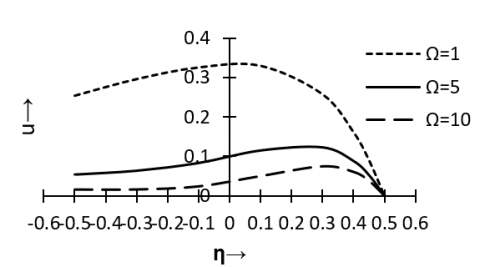Figure 2. Primary velocity distribution versus η for Pr=0.71, Sc=0.3, Re=1, N=1, M=1, P=5, h=1, m=1, Gr=1, Gm=1, λ=1, ω=5, t=0

## figure_3.png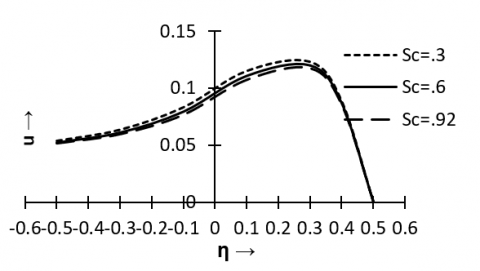Figure 3. Primary velocity distribution versus η for Pr=0.71, Re=1, N=1, M=1, P=5, h=1, m=1, Gr=1, Gm=1, λ=1, ω=5, Ω=5, t=0

An interesting behaviour of fluid velocity under solutal Grashof number $\mathrm{G}_{\mathrm{m}}$ is observed in Figure 4. It is observed in this Figure that the flow is accelerated due to solutal buyonacy force near the right wall and opposite trend of behaviour is marked near the left wall.

## figure_4.png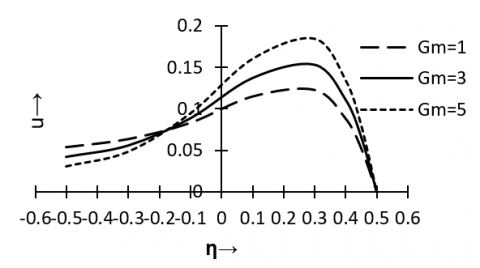Figure 4. Primary velocity distribution versus η for PR=0.71, Sc=0.3 Re=1, N=1, M=1, P=5, h=1, m=1, Gr=1, λ=1, Ω=5, t=0

The variations in the secondary velocity v versus $\Omega, G_{m}$ and $\eta$  are presented in Figures 5 and  6. It  is  inferred  from Figure 5 that there is a substantiall  fall  in  the  magnitude  of the  secondary  fluid  velocity  under  the  effect   of   rotation parameter $\Omega$  where as Figure 6 shows that an increase in solutal Grashof number $G_{\mathrm{m}}$  results in steady increase in the secondary velocity.

## figure_5.png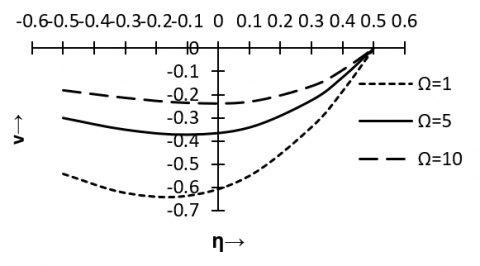Figure 5. Secondary velocity distribution versus η for Pr=0.71, Sc=0.3, Re=1, N=1, M=1, P=5, h=1, m=1, Gr=1, Gm=1, λ=1, ω=5, t=0

## figure_6.png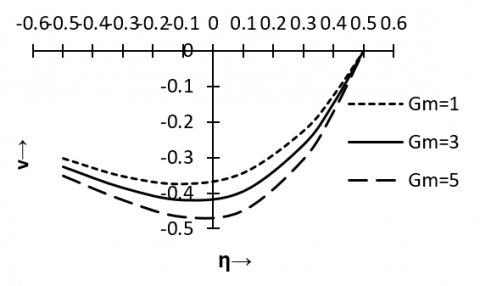Figure 6. Secondary velocity distribution versus η for Pr=0.71, Sc=0.3, Re=1, N=1, M=1, P=5, h=1, m=1, Gr=1, λ=1, ω=5, Ω=5, t=0

Figures 7 to 9 demonstrate the behaviour of concentration field under the effects $\lambda, \mathrm{S}_{\mathrm{c}}$ and $R_{e}$ . From these figures we see that there is steady fall in concentration for the increasing values of λ, $\mathrm{S}_{\mathrm{c}}$ and $\mathrm{R}_{\mathrm{e}}$ . In other words concentration boundary layer rises for enhancement of mass diffusivity and viscosity.

## figure_7.pngFigure 7. Concentration distribution versus η for Sc=0.3, Re=1, ω=5, t=0

## figure_8.png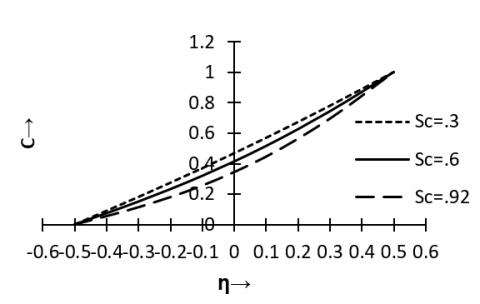Figure 8. Concentration distribution versus η for Re=1, λ=1, ω=5, t=0

## figure_9.png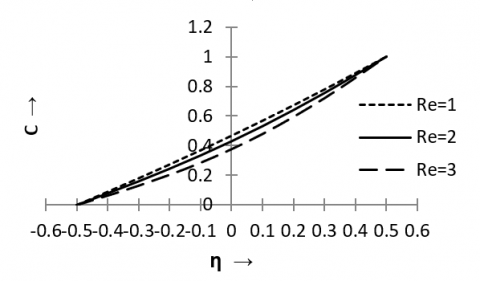Figure 9. Concentration distribution versus η for Sc=0.3, λ=1, ω=5, t=0

## figure_10.png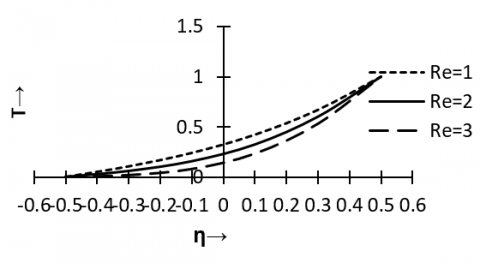Figure 10. Temperature distribution versus η for Pr=0.71, N=1, λ=1, ω=5, t=0

The effect of Reynolds number $\mathrm{R}_{\mathrm{e}}$  on temperature field against η is displayed in figure 10. It is marked from this figure that the temperature boundary layer decline with the increase of $\mathrm{R}_{\mathrm{e}}$ .

Figure 11 presents the variation of skin friction ζx due to primary velocity versus frequency parameter ω under the influence on $\mathrm{R}_{\mathrm{e}}$.The figure 11 shows wavy character of the skin friction ζx under ω. It is also observed in figure 11 that the magnitude of ζx rises as $\mathrm{R}_{\mathrm{e}}$  increases. We recall that $\mathrm{R}_{\mathrm{e}}$  increases means viscosity drag falls gradually as fluid friction increases. Further it is noticed that for large values ω the effect of $\mathrm{R}_{\mathrm{e}}$ on ζx is not so pronounced.

## figure_11.png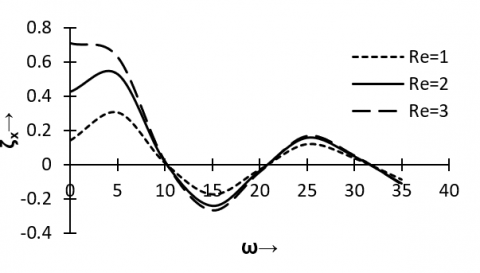Figure 11. Primary skin friction versus ω for Pr=0.71, Sc=0.3, N=1, M=1, P=5, h=1, m=1, Gr=1, Gm=1, λ=1, Ω=5, t=0.3

The effect of $\mathrm{R}_{\mathrm{e}}$  on ζy, the skin friction due to secondary velocity is demonstrated in figures 12. This figure shows that the change of behaviour of ζy under $\mathrm{R}_{\mathrm{e}}$  and ω are analogous to the corresponding behaviour of ζx under the same parameters. That is magnitude of ζy increases as $\mathrm{R}_{\mathrm{e}}$  increases. Further, figure 12 registers the fact that ζy is not influenced by $\mathrm{R}_{\mathrm{e}}$  for large ω.

## figure_12.pngFigure 12. Secondary skin friction versus ω for PR=0.71, Sc=0.3, N=1, M=1, P=5, h=1, m=1, Gr=1, Gm=1, λ=1, Ω=5, t=0.3

## figure_13.png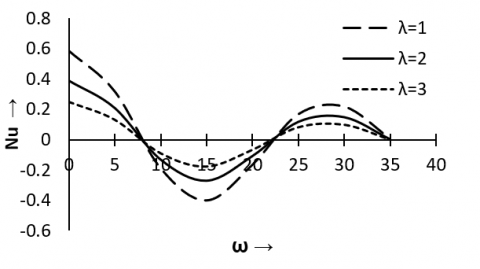Figure 13. Nusselt number versus ω for Pr=0.71, Re=1, N=1, t=0.3

The wavy character of behaviour of the Nusselt number Nu like ζx and ζy under ω are reflected in the figure 13. This figure depicts that the magnitude of Nusselt number rises as injection / suction parameter λ decreases. In other words injection / suction causes of heat transfer to decrease substantially.

Figures 14 and 15 illustrate the variation in Sherwood number under the effect of $\mathrm{S}_{\mathrm{c}}$ and $\mathrm{R}_{\mathrm{e}}$ . It is marked from this figures that curves are wavy as like figures 15 to 20 and for increasing values of $\mathrm{S}_{\mathrm{c}}$ or $\mathrm{R}_{\mathrm{e}}$ , magnitude of Shewood number Sh increases. In other words rate of mass transfer decreases for enhancement of mass diffusivity and viscosity. Further it is noticed that for small values of ω, the effect of $\mathrm{S}_{\mathrm{c}}$  and $\mathrm{R}_{\mathrm{e}}$  is almost unpronounced.

## figure_14.png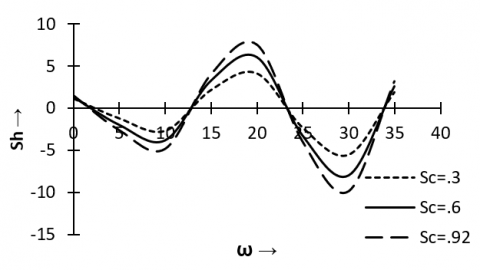Figure14. Sherwood number versus ω for Re=1, λ=1, t=0.3

## figure_15.png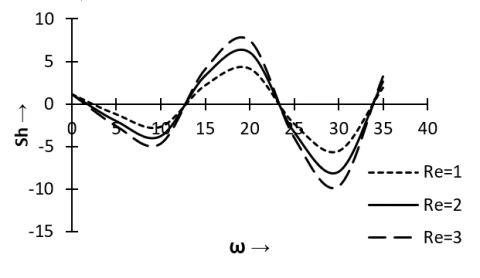Figure 15. Sherwood number versus ω for Sc=0.3, λ=1, t=0.3

5. Conclusions

1. High mass diffusivity accelerates the flow.

2. There is a substantial fall in the magnitude of the secondary velocity under the effect of rotation.

3. Thickness of the concentration boundary layer gets enhanced due to mass diffusivity as well as internal friction of the fluid.

4. The pressure gradient has significant contribution for increasing the viscous drag in the mean flow direction.

5. The magnitude of Sherwood number increases for increasing values of Sc or Re

6. The study of the present problem finds wide scope of applications in civil engineering, chemical engineering, a mechanical engineering, geophysics, astrophysics etc.

Nomenclature

$\vec{B}$               magnetic induction vector,

B0             magnetic inductance, Tesla

C0             mean concentration, K. mol. m-3

Cp             specific heat at constant pressure, J. kg-1. K-1

C'              dimensional concentration,  K. mol. m-3

D              molar mass diffusivity, m2. s-1

$\vec{E}$              electric field,

e               electron charge, ampere

Gm            solutal Grashof number,

Gr             thermal Grashof number,

g               acceleration due to gravity, m. s-2

h               slip flow parameter,

$\vec{J}$               current density vector,

k               thermal conductivity, W. m-1. K-1

M              Hartmann number,

m              Hall parameter,

Pr              Prandtl number,

p               pressure, N. m-2.

Pe              electron pressure,

Re             Reynolds number,

Sc              Schmidt number,

T0             mean temperature, K

T'              dimensional temperature, K

t'               time, sec

U              mean axial velocity, m. s-1

u'             x – component of $\vec{V}$, m. s-1

u              non dimensional velocity in x direction

v'              y – component of $\vec{V}$, m. s-1

v               non dimensional velocity in y direction

Wo            injection/suction velocity, m. s-1

$\vec{\Omega }$             angular velocity of the field,

${\Omega }'$           uniform angular velocity, sec-1

$\Omega$             rotation parameter,

$\rho$            density, kg. m-3

$\mu$            coefficient of viscosity, kg. (m. s)-1

$\upsilon$            kinematic viscosity,   m2. s-1

$\sigma$              electrical conductivity, (ohm. m)-1

${{\omega }_{e}}$            electron frequency, sec-1

${{\tau }_{e}}$            electron collision time, sec

${{\eta }_{e}}$           number density of electron, m-3

$\lambda$             injection/suction parameter,

${\omega }'$           dimensional frequency of oscillation, sec-1

$\omega$            frequency of oscillation.

$\beta$             volumetric coefficient of thermal expansion, K-1

$\bar{\beta }$             volumetric coefficient for solutal expansion, (K. mol)-1

References

 W. L. Cooper, V. W. Nee and K. T. Young, “Fluid mechanics of oscillatory and modulated flows and associated applications in heat and mass transfer,” Journal of Energy, Heat and Mass transfer, vol. 15, pp. 1-19, 1993.

 R. Muthucumaraswamy, “Effect of heat and mass transfer on flow past an oscillating vertical plate with variable temperature,” Int. J. of Appl. Math of Mech., vol.  4, no. 1, pp. 59-65, 2008.

 N. Ahmed and H. Kalita, “Oscillatory MHD free and forced convection flow  through a  porous medium in presence  of a heat source with variable suction,” International Journal of Heat and Technology, vol. 28, no. 1, pp.141-147, 2010

 L. Debnath, “Exact solutions of the unsteady hydrodynamic and hydro-magnetic boundary layer in rotating fluid system,” ZAMM, vol. 55, no. 7 – 8, pp. 431-438, 1975. DOI: 10.1002/zamm.19750550712.

 M. Guria, S. Das, R. N. Jana and S. K. Ghosh, “Oscillatory couette flow in the presence of an inclined magnetic field,” Meccannica, vol. 44, pp. 555-564, 2009. DOI: 10.1007/s11012 – 009 – 9195 – 1.

 E. M. Aboeldahab and E.M.E. Elabarbary, “Hall current effect on magneto hydrodynamic free convection flow past a semi-infinite vertical plate with mass transfer,” Int. J. Eng. Sci., vol. 39, pp. 1641-1652, 2001.

 B. C. Sarkar, S. Das and R. N. Jana, “Effects of hall currents and radiation on MHD free convection flow past an oscillating vertical plate with oscillatory plate temperature in a porous medium,” Bulletin Soc. Math. Services and Standards, vol. 1, pp. 06 – 32, 2012.

 C.L.M.H. Navier, “Memoire Surles du Movement des,” Mem Acad. Sci. Inst. France, vol. 1, no. 6, pp. 414 – 416, 1823.

 S. Yu and T. A. Ahem, “Slip flow heat transfer in rectangular micro channel,” International Journal of Heat and Mass Transfer, vol. 44, no. 22, pp. 4225 – 4234, 2002. DOI: 10.1016/s0017-9310(01)00075-8.

 O. D. Makinde and  E. Osalusi, “MHD steady flow in a channel with slip at the permeable boundaries,” Rom. J. Phys., vol. 51, no. 3 – 4, pp. 319-328, 2006.

 W. Marques, G. M. Kremer and F. M. Shapiro, “Couette flow with slip and jump boundary conditions,” Continumm Mech. Thermodynam, vol. 12, pp. 379-386, 2000.

 N.Ahmed and D. Kalita, “MHD oscillatory free convective flow past a vertical plate in slip-flow regime with variable suction and periodic plate temperature,” International Journal of Heat and Technology, vol. 26, no. 2, pp. 85-92, 2008.

 K. D. Singh and R. Pathak, “Effect of slip conditions and Hall current on an oscillatory convective MHD flow in a rotating vertical porous channel with thermal radiation,” Int. J. of Appl. Math and Mech, vol. 9, no. 12, pp. 60 – 77, 2013.